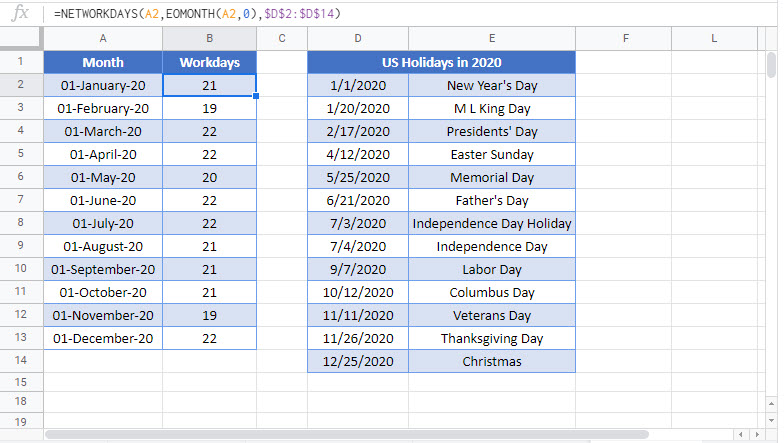# Number of Work / Business Days in Month – Excel & Google Sheets

This tutorial will demonstrate how to calculate the number of business days in a month in Excel and Google Sheets.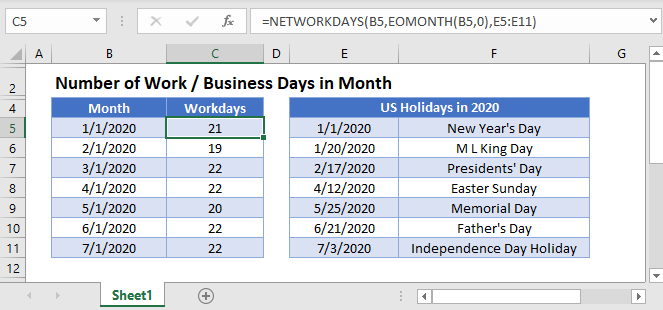## Number of Work Days in Excel

To calculate how many work days are in a month, you can use the NETWORKDAYS and EOMONTH Functions.

``=NETWORKDAYS(B3,EOMONTH(B3,0),E3:E15)``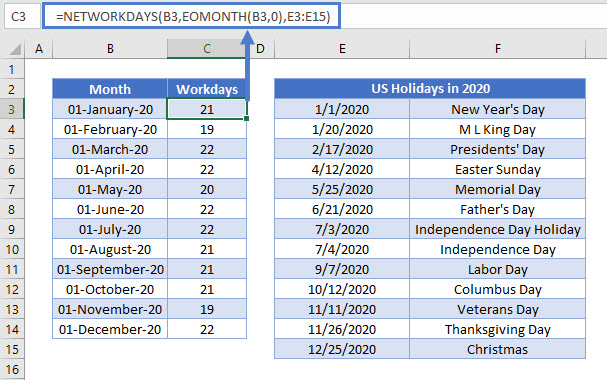We will walk through the formula in more detail below.

### EOMONTH Function

The EOMONTH Function returns the last day of the month given the start date and number of months.

``=EOMONTH(B3,0)``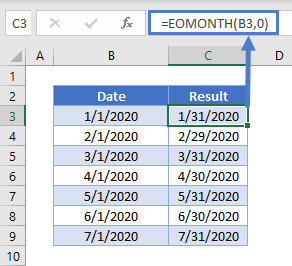In this example we use the EOMONTH Function (going zero months forward) to give the last day of the month in Column B.

### NETWORKDAYS Function

The NETWORKDAYS function returns the number of whole working days between the given start and end dates. It excludes weekends and any holidays that you specify.

``=NETWORKDAYS(B3,C3,E3:E15)``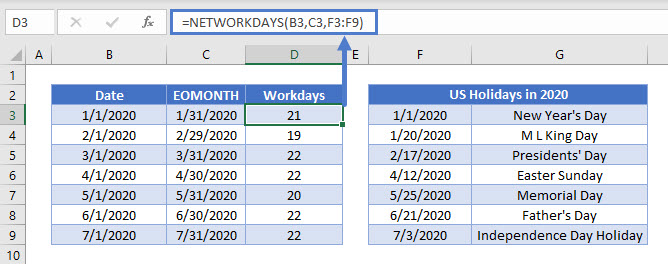Notice the holidays have been specified off to the side. You can list any number of holidays you like. Here’s an example of how the optional holidays work in the NETWORKDAYS function:

• January 2020 has 31 days total.
• 23 of the days in January are “work days” (Monday–Friday).
• There are 2 holidays listed for work days (one on a Thursday and one on a Monday) in January.

So, our formula gives us 21 (23 – 2) for January.

Combining the steps above gives us our original formula:

``=NETWORKDAYS(B3,EOMONTH(B3,0),E3:E15)``